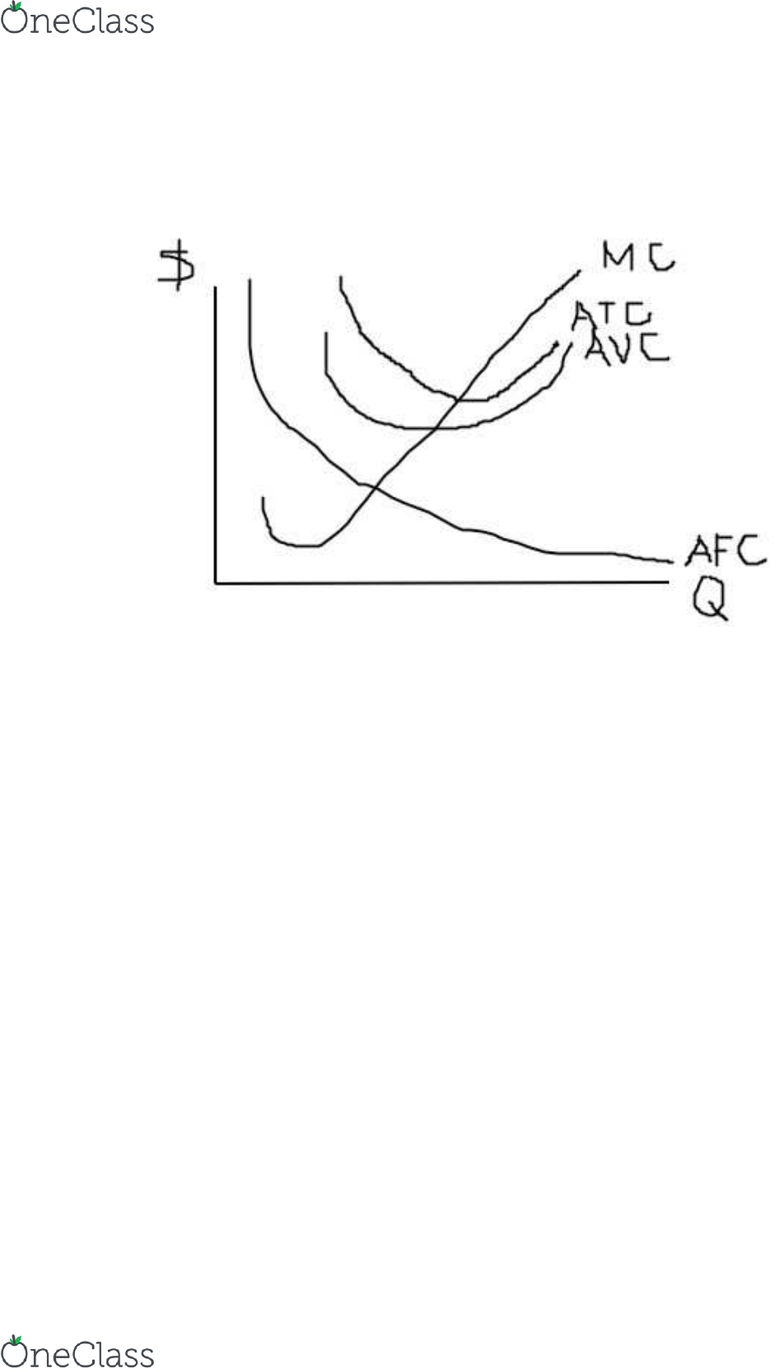# ECON 1 Lecture 21: ECON 1 11-10-2015

266 views3 pages
School
UCSD
Department
Economics
Course
ECON 1
Page:
of 3ECON 1
MP = Δ output / Δ input
MC = Δ cost / Δ output = Δ input * C (cost of input) / Δ output = C / MP
Chapter 6 Problem Set Firm Production & Cost Question 2
a.
AFC decrease over time
MC cuts ATC, AVC at their minimum
Distance between ATC and AVC decrease
b. Labor is input of production MC increase
c. AVC increase, AFC constant, ATC increase
d. Input constant and output increaseMP increaseMC decrease
π (profit) = Total Revenue – Total Cost
= P * Q – TFC – TVC (Q)
= P * Q – AFC * Q – AVC * Q
Chapter 6 Problem Set Profit Maximization Question 1
find more resources at oneclass.com
find more resources at oneclass.com
a.
b. 0 = P * Q – AFC * Q – AVC * Q
P – AFC – AVC = 0
P – ATC = 0
P = ATC
P = MC
P = MC = ATC (breakeven point)
MC = AVC (shutdown point)
find more resources at oneclass.com
find more resources at oneclass.com
c.
Blue area = profit
Chapter 6 Problem Set Profit Maximization Question 2
Chapter 6 Problem Set Profit Maximization Question 3
a. MC = Q2
AC = Q2 / 3
P* = 81
P = Q2
81 = Q2
Q* = 9
b. P* Q* = 81* 9 = 729
ATC = 92 / 3 = 27
TC = ATC * Q = 27 * 9 = 243
π = 729 – 243 = 486
c. Marginal productivity will drop
Chapter 6 Problem Set Profit Maximization Question 4
find more resources at oneclass.com
find more resources at oneclass.com

## Document Summary

Mc = cost / output = input * c (cost of input) / output = c / mp. Chapter 6 problem set firm production & cost question 2 a. Distance between atc and avc decrease: labor is input of production mc increase, avc increase, afc constant, atc increase d. (profit) = total revenue total cost. = p * q tfc tvc (q) = p * q afc * q avc * q. Chapter 6 problem set profit maximization question 1 a: 0 = p * q afc * q avc * q. Chapter 6 problem set profit maximization question 2. Chapter 6 problem set profit maximization question 3: mc = q2. Q* = 9: p* q* = 81* 9 = 729. Tc = atc * q = 27 * 9 = 243. = 729 243 = 486: marginal productivity will drop.